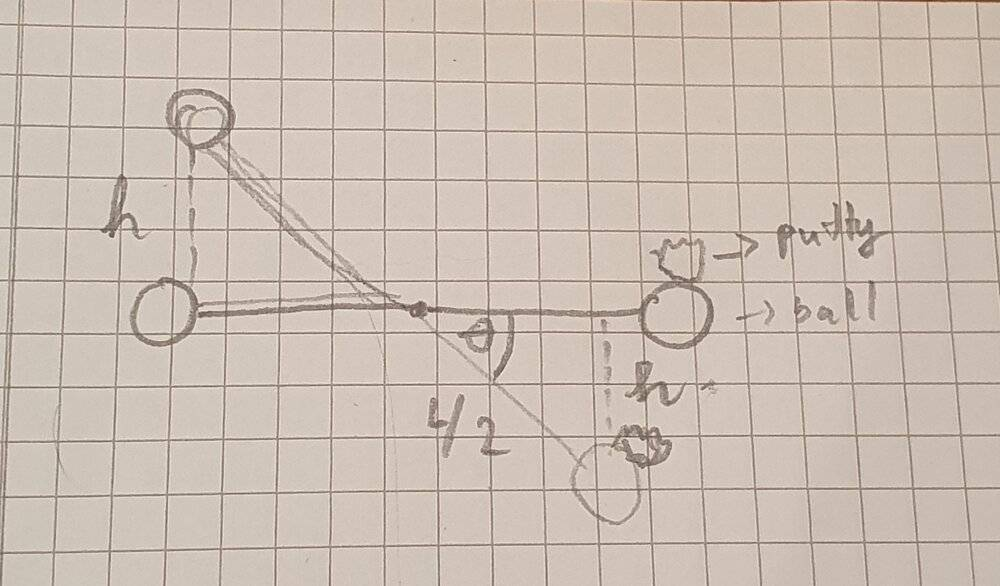# Rotational motion and angular momentum

hquang001
Homework Statement:
Two 2.00 kg balls are attached to the ends of a thin rod of length 50.0 cm and negligible mass. The rod is free to rotate in a vertical plane without friction about a horizontal axis through its center. With the rod initially horizontal, a 50.0 g wad of wet putty drops onto one of the balls, hitting it with a speed of 3.00 m/s and then sticking to it.
(a) What is the angular speed of the system just after the putty wad hits?
(b) What is the ratio of the kinetic energy of the system after the collision to that of the putty wad just before?
( c) Through what angle will the system rotate before it momentarily stops?
Relevant Equations:
$$L_{initial} = L_{final}$$
$$K_{initial} + PE_{initial} = K_{final} + PE_{final}$$
mball = 2 kg, mputty = 0.05 kg, L = 0.5 m, v = 3m/s
a) Moment of inertia : I = (2mball + mputty ). ¼ L^2 = 0.253125 kg.m^2

Linitial = Lfinal => mputty. v. r = I.ω => ω = (4.mputty.v.r) / I = 0.148 rad/s

b) K initial = 1/2 m v^2 = 0.225 J
K final = 1/2 Iω^2 = 2.85.10^(-3) J => Kfinal / Kinitial = 0.013

c) Using conservation of energy :K initial +PE initial = K final + PE final
1/2 I.ω^2 + 0 = 0 + (mball + mputty).g.(-h) + mball.g.h1/2 I.ω^2 = (2.05).g. (-sinΘ . L/2 ) + (2).g.(sinΘ . L/2)
=> 1/2 I.ω^2 = g.sinΘ . L/2 . (2 - 2.05) => Θ = -1.3°
I think the angle should be larger than 180 => 180° - (-1.3°) = 181°

I don't know if my answer for question c is correct or not

Last edited:

Homework Helper
Gold Member
2022 Award
It all looks right. It should definitely be 181.3°, of course.

•Steve4Physics
Homework Helper
Gold Member
2022 Award
Edit - typo's corrected.

If you release a simple pendulum (negligible friction/air resistance) what height does it reach on the other side, and why?

If you give the same pendulum a small downwards push at the moment of release, what can you say about the height it reaches on the other side, and why?

I get a very slightly different answer to you for part b). This could be caused by a small rounding error in your calculation. (Don't use rounded values in intermediate steps.) Your working isn't clear enough for me to check what you've done in detail but the method is OK.

As noted by @PeroK, you are asked through what angle the system rotates - not what angle it makes relative to its starting position.

•PeroK
hquang001
If you release a simple pendulum (negligible friction/air resistance) what height does it reach on the other side, and why?
I think it should reach the same height because there is no outside force. we can proof it by using conservation of energy
PE initial = PE final => mgh1 = mgh2 => h1 = h2
If you give the same pendulum a small downwards push at the moment of release, what can you say about the height it reaches on the other side, and why?
I get a very slightly different answer to you for part b). This could be caused by a small rounding error in your calculation. (Don't use rounded values in intermediate steps.) Your working isn't clear enough for me to check what you've done in detail but the method is OK.

- for part b). yes you're right. As i rounded the value of angular speed 0.148 to 0.15 rad/s so the answer is not quite correct. The final kinetic energy should be 2.77.10^(-3) J => the ratio is 0.0123

•Steve4Physics
Homework Helper
Gold Member
2022 Award
Does it [a pendulum] reach greater height than original height [if given an initial push] ? I'm not sure about this
If you are holding a pendulum (at an angle) and give it an initial push, it will reach a bigger height compared to merely releasing it. The extra gravitational potential energy equals the extra initial kinetic energy you gave it (conservation of energy).

Applying the same argument to the original problem, you should see that the rod must rotate more than 180º, not less.

And you've sorted out the rounding error. Yay!

•hquang001
$$L_i = mur = (2M + m)vr = L_f$$ $$\therefore v = \mu u, \ \text{where} \ \mu = \frac{m}{2M+m}$$
For part a): $$\omega = \frac{v}{r} = \frac{\mu u}{ r}$$
For part b): $$\frac{KE_f}{KE_i} = \frac{(2M+m)v^2}{mu^2} = \frac{1}{\mu}\mu^2 = \mu$$
•